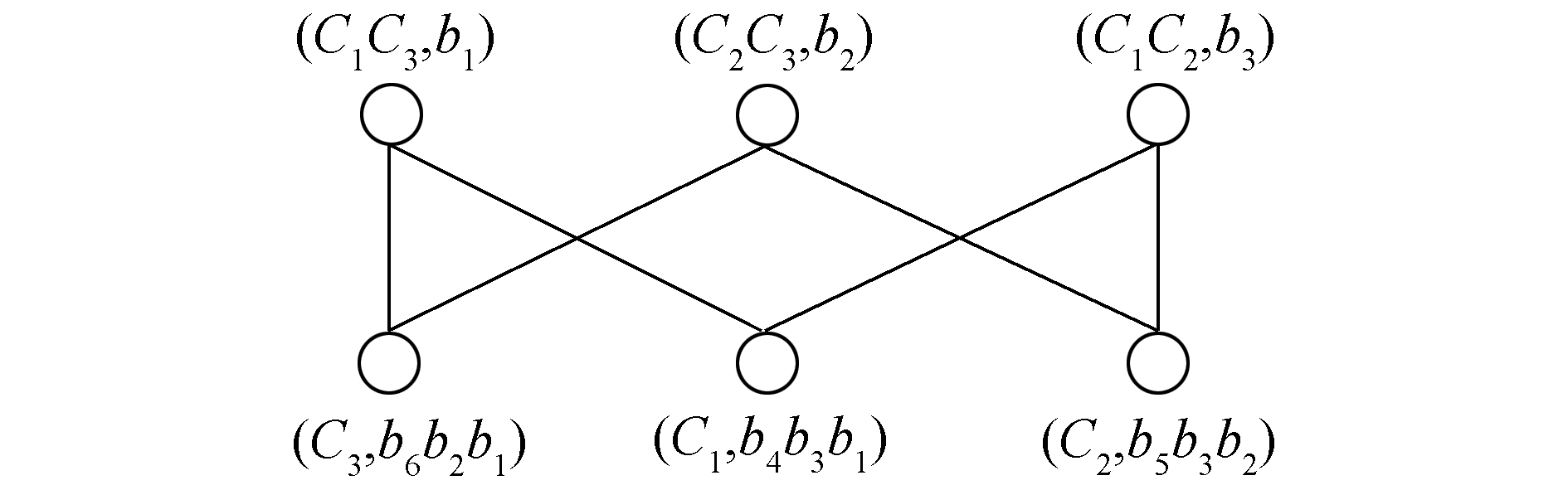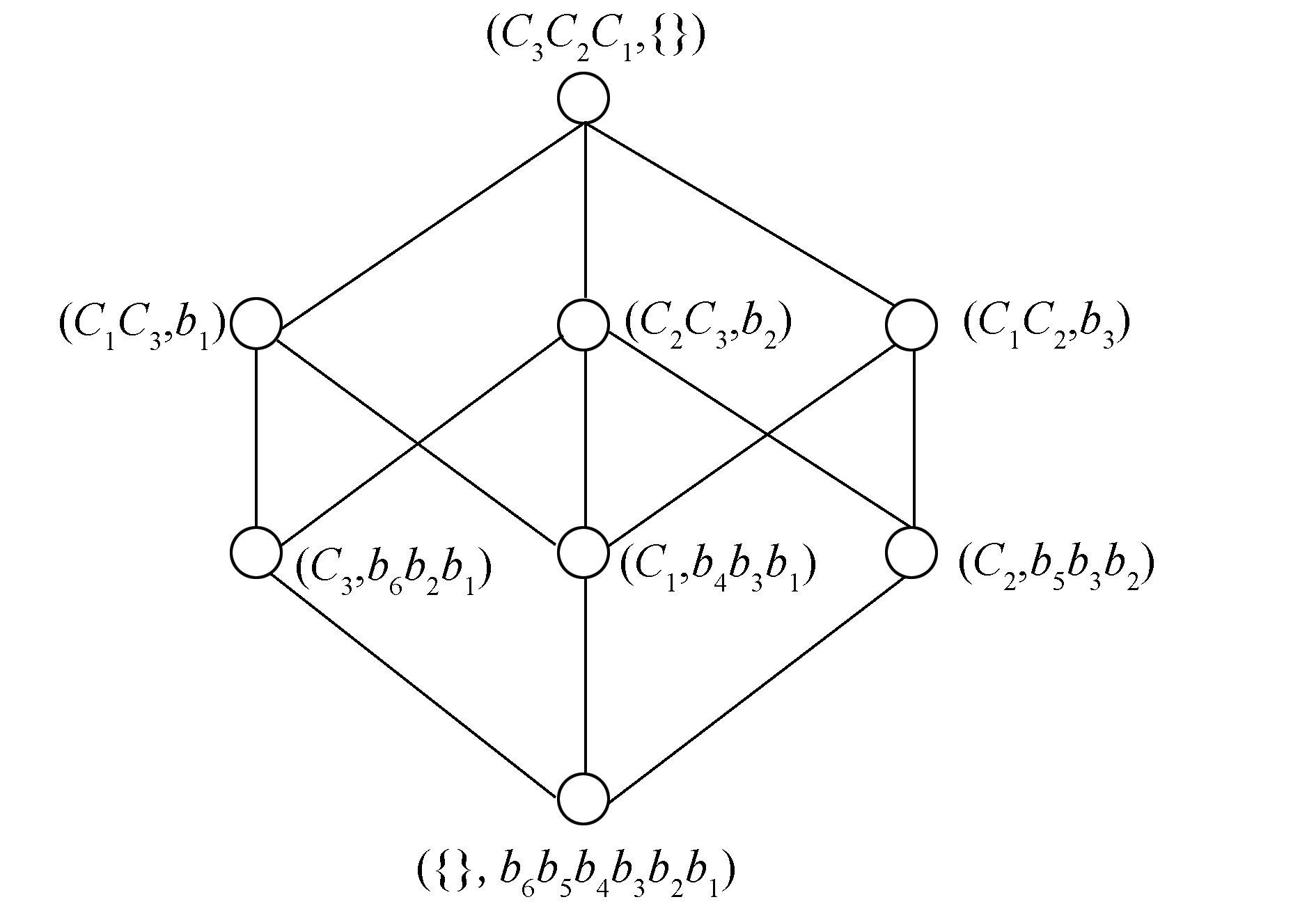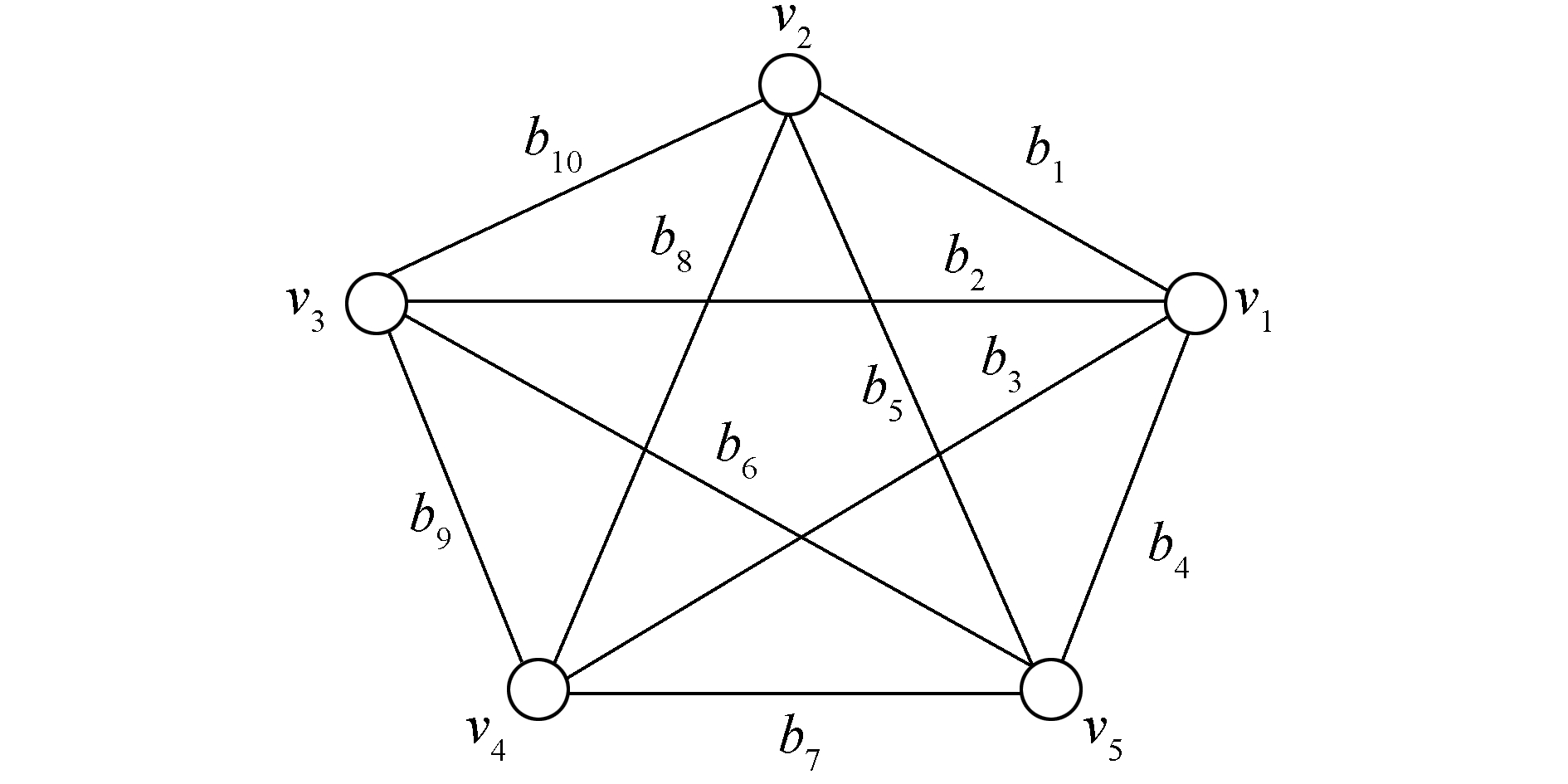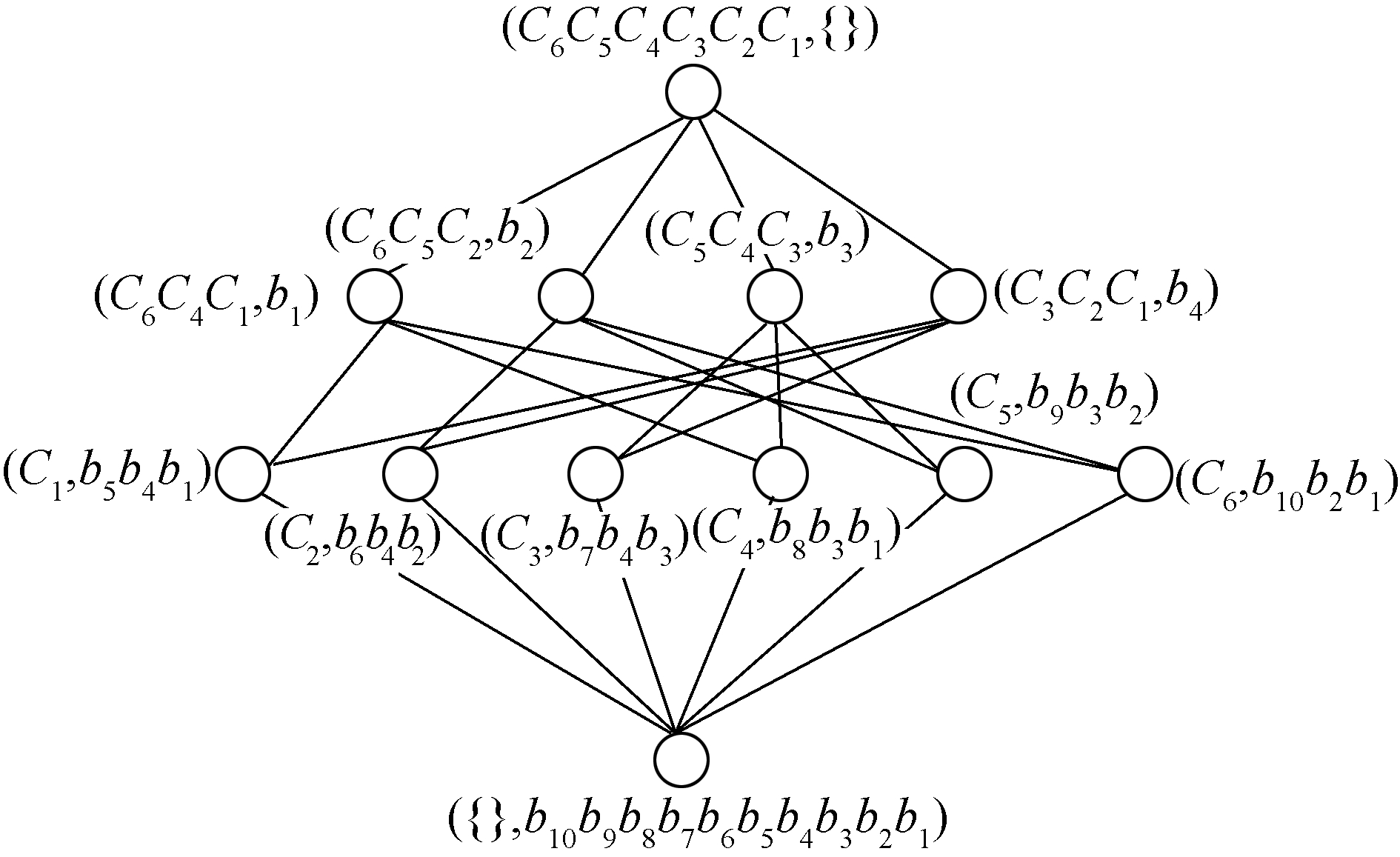﻿ 利用二元拟阵<i>K</i><i><sub>n</sub></i>图的一种建格方法
«上一篇文章快速检索 高级检索

 智能系统学报2017, Vol. 12Issue (3): 333-340  DOI: 10.11992/tis.2017040220

### 引用本文MAO Hua, SHI Ming. A constructive method of lattice using the Kn diagram of binary matroid[J]. CAAI Transactions on Intelligent Systems, 2017, 12(3): 333-340. DOI: 10.11992/tis.201704022.### 文章历史

A constructive method of lattice using the Kn diagram of binary matroid
MAO Hua, SHI MingSchool of Mathematics and Information Science, Hebei University, Baoding 071002, China
Abstract: Because of the complexity of traffic networks, it is difficult to directly analyze and deal with them. If some travelers wish to determine their travel strategy based on their preferences and habits, they should have a clear understanding of their travel plan. To address this problem, a traffic network, Kn model, was established in this study. It was used to elucidate how to transfer complex networks comprising loops or multiple edges to the Kn diagram. With the assistance of formal concept analysis, the corresponding Hasse diagram of the Kn model was provided. The Hasse diagram facilitates travelers to extract some attributes under certain preconditions, after which the travelers can easily continue their work. Hence, the study of the Kn diagram revealed that a triangle circle would form under some effects of specific multiple attributes. Thus, combining with the standard definition of the matrix for binary matroids, a special formal context was obtained. According to the particularity of the formal context, an algorithm was proposed based on the binary matroids for the Kn diagram. Utilizing an example, the feasibility of the proposed method was proven. Because the model is universal, the discussions of this research can be extended to other fields with similar formal context.
Key words: binary matroid    standard matrix representative    Kn diagram    bipartite graph    graph theory    concept lattice    formal context    Hasse diagram

1 预备知识

1)B′={aG| ∀bB, aIb}, A′={bM | ∀aA, aIb};

2)AA″, BB″;

3)$(\underset{j\in J}{\mathop{\cup }}\, {{A}_{j}}{)}'=\text{ }\underset{j\in J}{\mathop{\cap }}\, {{A}_{j}}^{\prime }, (\text{ }\underset{j\in J}{\mathop{\cup }}\, {{B}_{j}}{)}'=\underset{j\in J}{\mathop{\cap }}\, {{B}_{j}}^{\prime }$

 \begin{align} & {{\vee }_{j\in J}}({{A}_{j}}, {{B}_{j}})=\left( {{\left( \underset{j\in J}{\mathop{\cup }}\, {{A}_{j}} \right)}^{\prime \prime }}, \underset{j\in J}{\mathop{\cap }}\, \text{ }{{B}_{j}} \right) \\ & {{\wedge }_{j\in J}}({{A}_{j}}, {{B}_{j}})=\left( \underset{j\in J}{\mathop{\cap }}\, \text{ }{{A}_{j}}, (\underset{j\in J}{\mathop{\cup }}\, {{B}_{j}}{)}'' \right) \\ \end{align}

2 模型的建立

1) 对于两个顶点之间具有多重边的情况，选择其中权值最小的边。

2) 当两个顶点之间的权值一样时，任意选择其中一条边，固定下来，成为最熟悉的一条道路。

3) 对于带环图的这种情况，因为实际问题考虑的是对于一个工厂的运输，自身运输没有任何实际的意义。例如：如果是一家超市，自身对于自身运输也没有意义，所以不予考虑。

2.1 问题的提出

2.2 模型的条件

2.3 问题的分析

2.4 模型的解决 2.4.1 Kn图的建格模型表 1 顶点数与基最小圈的规律 Tab.1 Vertex number and the basis of the smallest circle of the law表 2 Kn图的形式背景L(Okn, Pkn, Ikn) Tab.2 Formal context L(Okn, Pkn, Ikn) of Kn diagram

L(O, P, I)最大元为A即第一层，对任意XL(O, P, I)|{A}，设AX的距离为n。也就是，当区间[X, A]⊆ L(O, P, I)最长链的长度为r，(r=1, 2, 3, …, n)，则设X为第m层，m=r+1, 记第m层为m=L(m)。设概念格L(O, P, I)的Hasse示图记为H(L)。由表 2发现，当n=4时，根据L(Okn, Pkn, Ikn)={(P′, P)}得对应的概念为(C3C2C1, {})∈L(1)；(C1C3, b1)，(C3C2, b2)，(C1C2, b3)∈L(2)；(C3, b6b1b2)，(C1, b4b1b3)，(C2, b5b2b3)∈L(3)；({}, b6b5b4b3b2b1)∈L(4)

2) 根据定义9含两个基的基最小圈最多有一条重边。图 1 K4图的二部图 Fig.1 Bipartite graph of K4 diagram图 2 K4图的概念格 Fig.2 Concept lattice of K4 diagram

2.4.2 算法过程

1)C：={P′, P}初始化属性集合B=Ø。

2) 找第2层的概念，根据规律知道，当完全图为Kn时，顶点数为n，与基顶点相连的边为n-1, 即第2层的概念为n-1个，根据属性b1, b2, …，bn-1找到所对应的基最小圈对象。

3) 找第3层的概念，根据完全图Kn的特点，基最小圈的个数为$\frac{n\left( n-1 \right)}{2}-\left( n-1 \right)$，即第3层有概念$\frac{n\left( n-1 \right)}{2}-\left( n-1 \right)$个，由对象C1C2, …，${{C}_{\frac{n\left( n-1 \right)}{2}-\left( n-1 \right)}}$，找到所对应的属性。

4) 第1层中的元(概念)，与且仅与第2层的每一个元分别连线，第4层的元与且仅与第3层的每一个元分别连线，第2层的元与第3层的元之间的连线关系可以根据定理2进行。这样就可以得到所有应该存在的连线。即根据定义6和引理2的偏序关系(C1, b1)≤(C2, b2)⇔C1C2(⇔b2b1)，满足概念格子概念-父概念的关系，把第1层的概念(O, {})与第2层的概念建立连线，第4层的概念({}, P)与第3层概念建立连线加入到步骤2) 和3) 组成的二部图中。

2.4.3 算法的复杂度

3 应用实例

 $\boldsymbol{A}=\begin{matrix} {} \\ {{v}_{2}} \\ {{v}_{3}} \\ {{v}_{4}} \\ {{v}_{5}} \\ \end{matrix}\left[\begin{matrix} {{b}_{1}} & {{b}_{2}} & {{b}_{3}} & {{b}_{4}} & {{b}_{5}} & {{b}_{6}} & {{b}_{7}} & {{b}_{8}} & {{b}_{9}} & {{b}_{10}} \\ 1 & 0 & 0 & 0 & 1 & 0 & 0 & 1 & 0 & 1 \\ 0 & 1 & 0 & 0 & 0 & 1 & 0 & 0 & 1 & 1 \\ 0 & 0 & 1 & 0 & 0 & 0 & 1 & 1 & 1 & 0 \\ 0 & 0 & 0 & 1 & 1 & 1 & 1 & 0 & 0 & 0 \\ \end{matrix} \right]$图 3 K5图 Fig.3 K5 diagram

 ${{\boldsymbol{A}}^{*}}=\begin{matrix} {} \\ {{C}_{1}} \\ {{C}_{2}} \\ {{C}_{3}} \\ {{C}_{4}} \\ {{C}_{5}} \\ {{C}_{6}} \\ \end{matrix}\left[\begin{matrix} {{b}_{1}} & {{b}_{2}} & {{b}_{3}} & {{b}_{4}} & {{b}_{5}} & {{b}_{6}} & {{b}_{7}} & {{b}_{8}} & {{b}_{9}} & {{b}_{10}} \\ 1 & 0 & 0 & 1 & 1 & 0 & 0 & 0 & 0 & 0 \\ 0 & 1 & 0 & 1 & 0 & 1 & 0 & 0 & 0 & 0 \\ 0 & 0 & 1 & 1 & 0 & 0 & 1 & 0 & 0 & 0 \\ 1 & 0 & 1 & 0 & 0 & 0 & 0 & 1 & 0 & 0 \\ 0 & 1 & 1 & 0 & 0 & 0 & 0 & 0 & 1 & 0 \\ 1 & 1 & 0 & 0 & 0 & 0 & 0 & 0 & 0 & 1 \\ \end{matrix} \right]$表 3 K5图的形式背景 Tab.3 Formal context of K5 diagram

1)C：={P′, P}初始化属性集合B=Ø。

2) 找第2层的概念，根据属性b1b2b3b4b5b6找到所对应的基最小圈对象C1C4C6C2C5C6C3C4C5C1C2C3得到概念(C1C4C6b1)、(C2C5C6b2)、(C3C4C5, b3)、(C1C2C3, b4)。

3) 找第3层的概念，根据完全图Kn的特点，由对象C1C2C3C4C5C6找到所对应的属性b1b4b5b2b4b6b3b4b7b1b3b8b2b3b9b1b2b10得到概念(C1, b1b4b5)、(C2b2b4b6)、(C3, b3b4b7)、(C4, b1b3b8)、(C5b2b3b9)、(C6, b1b2b10)。

4) 把第1层和第4层概念(O, {})和({}, P)，根据定义6和引理2的偏序关系(C1, b1)≤(C2, b2)⇔C1C2(⇔b2b1)，对满足概念格子概念-父概念的关系，把第1层的概念(O, {})与第2层的概念建立连线, 第4层的概念({}, P)与第3层概念建立连线加入到步骤2) 和3) 组成的二部图中，得到概念格的Hasse示图，如图 4图 4 K5图的概念格 Fig.4 Concept lattice of K5 diagram

4 结束语

  左锋. 经济学视野中大城市经济圈的形成与发展—论中国三大城市经济圈的现状及其发展[J]. 华东师范大学学报:哲学社会科学版, 2002, 34(5): 89-95. ZUO Feng. The formation and development of a metropolitan economic circle in the perspec-tive of economics[J]. Journal of east cluna normal university:philosophy and social sciences, 2002, 34(5): 89-95. (0)  胡一竑. 基于复杂网络的交通网络复杂性研究[D]. 上海: 复旦大学, 2008: 1-121. HU Yihong. Analysis of complex transportation networks[D].Shanghai: Fudan University, 2008: 1-121. http://d.wanfangdata.com.cn/Thesis/Y1965645 (0)  刘佳. 复杂网络中最短路径问题的优化算法研究[D]. 太原: 太原科技大学, 2007: 1-30. LIU Jia. Research on the algorithm for shortest path problem in complicated network[D]. Taiyuan: Taiyuan University of Science and Technology, 2007: 1-30. http://cdmd.cnki.com.cn/Article/CDMD-10109-2007127989.htm (0)  严寒冰, 刘迎春. 基于GIS的城市道路网最短路径算法探讨[J]. 计算机学报, 2000, 23(2): 210-215. YAN Hanbing, LIU Yingchun. A new algorithm for finding shortcut in a city's road net based on GIS technology[J]. Chinese journal of computers, 2000, 23(2): 210-215. (0)  谢政, 李建平. 网络算法与复杂性理论[M]. 北京: 国防科技大学出版社, 1995: 120-140. (0)  LAM W H K, LI Zhichun, HUANG Haijun, et al. Modeling time-dependent travel choice problems in road networks with multiple user classes and multiple parking facilities[J]. Transportation research part B: methodological, 2006, 40(5): 368-395. DOI:10.1016/j.trb.2005.05.003 (0)  GAO Song, Chabini I. Optimal routing policy problems in stochastic time-dependent networks[J]. Transportation research parB: methodological, 2006, 40(2): 93-122. DOI:10.1016/j.trb.2005.02.001 (0)  于德新, 杨薇, 杨兆升. 重大灾害条件下基于GIS的最短路径改进算法[J]. 交通运输工程学报, 2011, 11(4): 123-126. YU Dexin, YANG Wei, YANG Zhaosheng. Shortest path improved algorithm based on GIS under large-scale disaster[J]. Journal of traffic and transportation engineering, 2011, 11(4): 123-126. (0)  陈京荣. 交通网络路径选择及应用研究[D]. 兰州: 兰州交通大学, 2009: 1-43. CHEN Jingrong. Research on route choice and its application in traffic networks[D]. Lanzhou: Lanzhou Jiaotong University, 2009: 1-43. http://cdmd.cnki.com.cn/Article/CDMD-10732-2010235726.htm (0)  GANTER B, Wille R. Formal concept analysis: mathematical foundations[M]. New York: Springer, 1999. (0)  王树禾. 图论[M]. 北京: 科学出版社, 2009. (0)  BONDY JA. Graph theory[M]. Berlin: Springer Press, 2008. (0)  刘桂珍, 陈庆华. 拟阵[M]. 长沙: 国防科技大学出版社, 1994. (0)  吉庆兵. 二元拟阵码及其对偶码[J]. 重庆师范学院学报:自然科学版, 2001, 18(4): 48-50. JI Qingbing. Binary matroid codes and their dual codes[J]. Journal of Chongqing normal university:natural science edition, 2001, 18(4): 48-50. (0)  马对霞, 林姿琼, 祝峰. 拟阵在网络安全中应用[J]. 小型微型计算机系统, 2015, 36(8): 1858-1860. MA Duixia, LIN Ziqiong, ZHU Feng. Application of matroids on network security[J]. Journal of Chinese computer systems, 2015, 36(8): 1858-1860. (0)  毛华. 拟阵与概念格的关系[J]. 数学进展, 2006, 35(3): 361-365. MAO Hua. The relation between matroid and concept Lattice[J]. Advances in mathematics, 2006, 35(3): 361-365. (0)  毛华, 李斌. 等价关系约束属性的形式概念分析[J]. 计算机工程与应用, 2010, 46(36): 158-160. Mao Hua, LI Bin. Formal concept analysis of attributes by equivalent relation[J]. Computer engineering applications, 2010, 46(36): 158-160. DOI:10.3778/j.issn.1002-8331.2010.36.043 (0)  李立峰, 刘三阳, 罗清君. 弦二部图的概念格表示[J]. 电子学报, 2013, 41(7): 1385-1388. LI Lifeng, LIU Sanyang, LUO Qingjun. Representing chordal bipartite graph using concept lattice theory[J]. Chinese journal of electronics, 2013, 41(7): 1385-1388. (0)  李立峰. 链图的概念格表示[J]. 计算机科学, 2014, 41(2): 264-266. LI Lifeng. Chain graph and their concept lattice representation[J]. Computer science, 2014, 41(2): 264-266. (0)  MAO Hua. A graph-theoretic method to representing a concept lattice[J]. Applied mathematics and information sciences, 2014, 8(2): 553-561. DOI:10.12785/amis/080213 (0)  MAO Hua. Characterizing trees in property-oriented concept lattices[J]. Armenian journal of mathematics, 2016, 8(2): 86-95. (0)  王海英, 黄强, 李传涛, 等. 图论算法及其MATLAB实现[M]. 北京: 北京航空航天大学出版社, 2010: 1-38. (0)  李娜. 形式概念分析中若干算法的改进与实现[D]. 北京: 中央民族大学, 2007: 1-15. LI Na. Improvement and implementation of several algorithms about formal concept analysis[D]. Beijing: Minzu University of China, 2007: 1-15. http://cdmd.cnki.com.cn/Article/CDMD-10052-2007071298.htm (0)